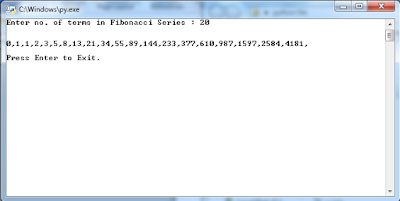## Tuesday, 29 May 2018

### To demonstrate use of slicing in string using python programming.

``` #Slicing in String print("SI = Starting index; EI = Ending index") nrStr = "I am Narendra Rajpoot." print("Length of Streing : ",len(nrStr)) print("String : ",nrStr) print("Charcter at index 3 : ",nrStr) print("String [SI = 3; EI = end of the sting] : ",nrStr[3:]) print("String [SI = 3; EI = 13] : ",nrStr[3:13]) print("String [SI = 0; EI = 10] ",nrStr[:10]) print("String [SI = 3; EI = 12; charcter differnce = 2]",nrStr[3:12:2]) input("\n\nPress Enter to exit.") ```

### To print ‘n terms of Fibonacci series using iteration using python programming.

``` n = int(input("Enter no. of terms in Fibonacci Series : ")) fTerm = 0 nTerm = 1 print("\n") for i in range(n):     if i<2:         print(i,end=",")     else:         temp = nTerm         nTerm = fTerm + nTerm         print(nTerm,end=",")         fTerm = temp input("\n\nPress Enter to Exit.") ```### To find all prime numbers within a given range using python programming.

``` # To find all prime numbers within a given range. import math n1 = int(input("Enter lower limit of range : ")) n2 = int(input("Enter upper limit of range : ")) print("\n") if n1==0:     n1+=1 if n1>0:     for i in range(n1,n2):         flag=0         sqr = math.sqrt(i)         for j in range(2,int(sqr)+1):             if j!=0:                 if (i%j)==0.0:                     flag=1                     break         if flag==0:             print(i,end=",") else:     print("Pleae enter positive limit: ") input("\n\nPress Any Key to Closse.") ```## Featured post

### To add 'ing' at the end of a given string (length should be at least 3). If the given string already ends with 'ing' then add 'ly' instead. If the string length of the given string is less than 3, leave it unchanged. <br/>Sample String : 'abc' <br/> Expected Result : 'abcing' <br/> Sample String : 'string'

#To add 'ing' at the end of a given string (length should be at least 3). If the #given string already ends with 'ing' then...